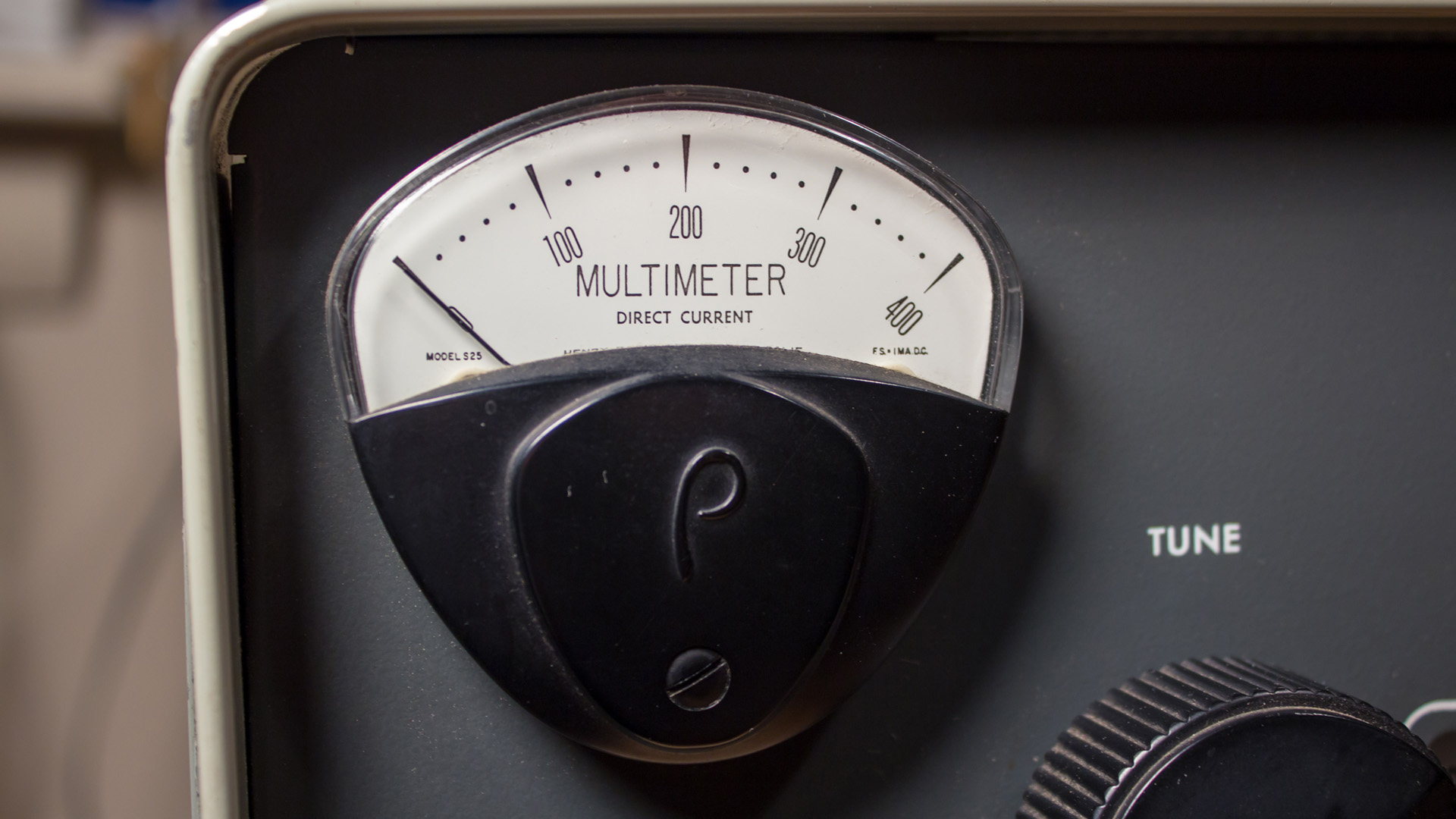Henry RF Amplifier Tear-drop Met

Henry RF Amplifier

Tear-Drop MetersHenry 2K

The original Henry Radio tear-drop meters are no longer manufactured. I have been asked this question many many many times.  You may find an original Henry meter on ebay, qrz or qth for sale, however if you do, be ready to pay up to and over \$200+ for one.

My suggestion would be to look on ebay or your local ham swap fest for an old tear-drop AC/DC volt or MA panel meter. They show up from time to time. They were commonly used in power plants and power distribution substations years ago. Don’t worry about what the meter measures or it's face scale, just purchase the meter

Once you have new replacement meter in-hand, dissemble it. Remove any shunt and multiple resistors inside the meter case and swap the scale face plate with the one from original bad Henry meter (Ip = 0-1A).  Use a DVM and identify the coil resistance. Then use the following information to determine the correct shunt and multiplier resistors. If you’re lucky and the coil is 50 ohms with 1ma full scale,  the shunt will be .2 ohms and the multiplier will 150 ohms.

Current Shunts: are a different beast all together. Assuming that you understand some principals of resistance in parallel this should be rather simple, but just to remind you, current will travel along the path of least resistance. Two resistors, of the same value, will equally share the current that travels through them, however if the values are not equal, current will take the SHORT path through the circuit. This is a SHUNT and in simple terms if we want a meter of 0-200 MA to read 0-2 AMP, we allow 1800 mA (1.8 AMP) to pass around the meter and allow 200 MA to pass through the METER.

The formula looks like this:R shunt = R meter / (n - 1) - Where R shunt is our meter shunt resistance, R meter is the inherent meter resistance and "n" is the multiplier. In our example the multiplier "n" is 5A divided by 1 mA or 5 / 0.001 which of course equals 5000. So (n - 1) must be 5,000 - 1 = 4,999. In this example the internal resistance of our meter is 60 OHMS.

R shunt = 60 / (4999) = 0.012 ohms -
Remember
the SHUNT resistor you choose must be capable of carrying the current you are trying to measure. The best rule of thumb is twice the value of the movement you are engineering. In this example 5A so a SHUNT of 10 AMP would protect the meter in case of over current issues, but only momentarily. A small 1 ohm resistor may be placed in series with the meter as a FUSE, however the value must be small and it should be considered when doing the math.

Most SHUNTS are made of COPPER WIRE. However we can use more exotic resistant type wire like that found in a TOASTER or HAIR DRYER. This wire works very well, but in a constant current application these wires will HEAT and cause several problems, including range changes as the wire expands and changes value. They will work in pulse type applications but you must be aware that there can be changes in readings as the wire temperature changes.

You can use CARBON resistors too, but generally in small current applications, where you are reading low current and you want to offer a multi-meter circuit using a rotary switch.

Henry RF Amplifier Information Center Web Site